Share
Explore

# f23 Mad 6135 Course Summary Documentf23 Mad 6135 Course Summary Document

### Learning Resources:

See Moodle for Lab and Lecture Worksheets:

### Mindmap: Use this as Study Cards

Concepts:
The skills to build web applications using:
HTML with BOM, Chromium Dev Tools
JavaScript:
In the Browser: COM
In Node: COM : Build server side JavaScript Applications (Jokes API)
JSON: a box into which you can package Data to be shipped around over a TCP/HTTP PIPE.
The BOM gives us hooks onto HTML DOM NODES which we can plug into with JavaScript APIS.
Thought Question:
What are the qualities of an HTML DOM NODE which we plug inth with JS APIs to entergize the operation of the HTML DOM of Nodes?
Attributes: <img src= <font size=
events: make nodes to be sources, or listeners to, events
Making nodes to listeners to events: how do we identify those nodes to receive:### class

id
html element nameCreating an HTML page that demonstrates event handling, selectors, and dynamic styling using CSS is a great way to learn how to manipulate the DOM. Here's a detailed example of such a page:
`This HTML page creates a simple calculator with two input fields for numbers, a select dropdown for selecting the operation, and a button to perform the calculation. `
`When you click the "Calculate" button, the result is displayed, and the button's color changes randomly. You can further customize the styles and functionality as needed.`

### <!DOCTYPE html> <html> <head> <style> body { font-family: Arial, sans-serif; }

.container { text-align: center; margin-top: 100px; }
input[type="number"] { padding: 10px; margin: 10px; width: 80px; }
select, button { padding: 10px; }
#result { font-size: 24px; margin-top: 20px; }
/* CSS for changing colors on button click */ .change-color { background-color: #3498db; color: white; }
.change-color:hover { background-color: #2c3e50; } </style> </head> <body> <div class="container"> <h2>Simple Calculator</h2> <input type="number" id="num1" placeholder="Enter a number"> <select id="operator"> <option value="add">Add</option> <option value="subtract">Subtract</option> <option value="multiply">Multiply</option> <option value="divide">Divide</option> </select> <input type="number" id="num2" placeholder="Enter another number"> <button id="calculate" class="change-color">Calculate</button> <p id="result"></p> </div>
<script> // Function to perform the calculation and update the result field function performCalculation() { const num1 = parseFloat(document.getElementById('num1').value); const num2 = parseFloat(document.getElementById('num2').value); const operator = document.getElementById('operator').value; let result;
switch (operator) { case 'add': result = num1 + num2; break; case 'subtract': result = num1 - num2; break; case 'multiply': result = num1 * num2; break; case 'divide': if (num2 !== 0) { result = num1 / num2; } else { result = 'Division by zero is undefined'; } break; }
const resultField = document.getElementById('result'); resultField.textContent = `Result: \${result}`; }
// Event listener for the Calculate button click const calculateButton = document.getElementById('calculate'); calculateButton.addEventListener('click', performCalculation);
// Event listener to change colors on button click calculateButton.addEventListener('click', () => { const colors = ['#e74c3c', '#27ae60', '#f1c40f', '#3498db']; const randomColor = colors[Math.floor(Math.random() * colors.length)]; calculateButton.style.backgroundColor = randomColor; }); </script> </body> </html>

### Review exercises:

Ways to create Classes and Objects
done: prototypical Constructor
Inheritance, Polymorphism, object composition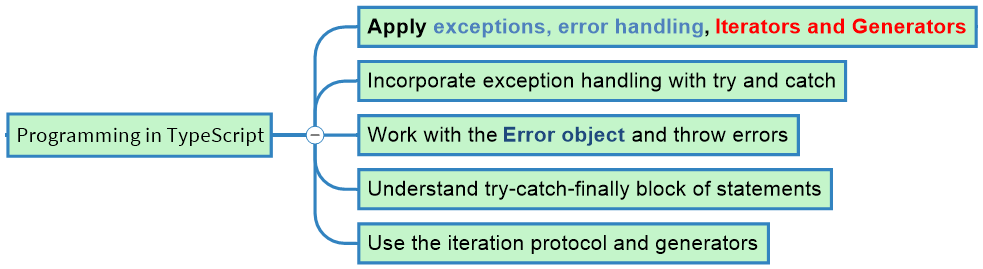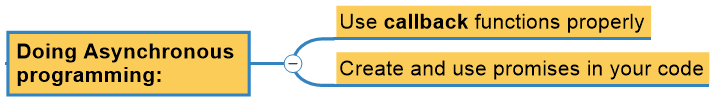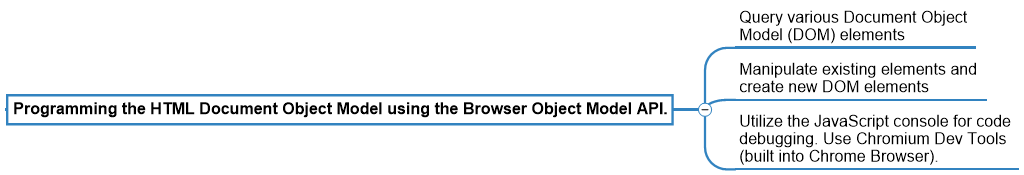### Practice Questions:

Write JavaScript or TypeScript code to illustrate how a member of a Module may act in the role of either a
data variable
or
a function.
Write code to illustrate how this would be expressed.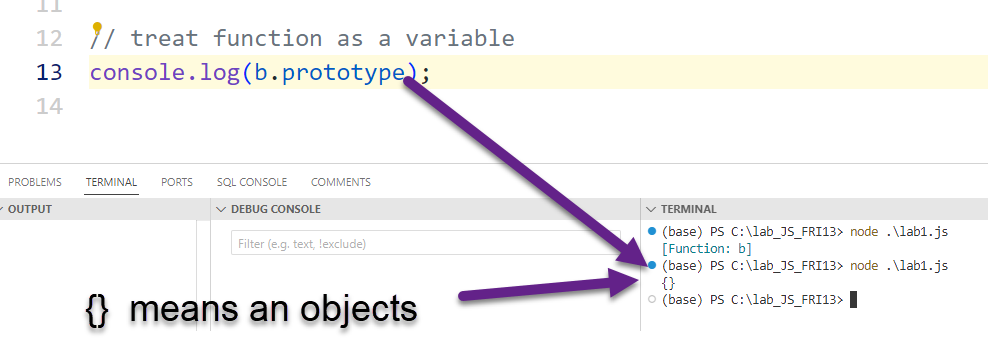### JavaScript Prototypical Constructors:

In JavaScript, every object has a prototype.
Recall: JS started out life as a simple scripting language. JavaScript later acquired the ability to be Object-Oriented, by introducing the Prototype Object.
Prototypes allow objects to inherit properties and methods from other objects, making it an essential part of the language.
When you create an object using a constructor function, that function's prototype becomes the prototype of the object you're creating.

### Treating Variables as Functions:

You can treat variables as functions in JavaScript, mainly by assigning functions to variables. In your code, you did precisely that with the function b.
`javascriptCopy code``let a = 1; // `a` is a variable`
`function b() {`` return 2;``} // `b` is a function`
`let whatami = (b.prototype); // You can access the `prototype` property of `b` (a function)``console.log(whatami); // Output will show the prototype of `b``
`The key point here is that everything in JavaScript has a Prototype, assigned by the JavaScript Language Server.`
Here, b is a function, and you access its prototype, treating it as a variable.

### Treating Functions as Variables:

Functions can also be treated as variables. You can assign functions to variables and pass them around like any other value.
`javascriptCopy code``let a = 1; // `a` is a variable`
`let b = function() {`` return 2;``}; // `b` is a variable storing a function`
`let result = b(); // You can call `b` like a function``console.log(result); // Output will be 2`

### Modifying Prototypes to Change Object Behavior:

“If every object has a prototype... What happens if I Modify an Object’s prototype AFTER I CREATE THE OBJECT?
You can add properties and methods to an object's prototype to change the behavior of objects created from that constructor.
`function Person(name) {`` this.name = name;``}`
`// Adding a method to the prototype``Person.prototype.greet = function() {`` return `Hello, my name is \${this.name}`;``};`
`let person1 = new Person('Alice');``let person2 = new Person('Bob');`
`console.log(person1.greet()); // Output: "Hello, my name is Alice"``console.log(person2.greet()); // Output: "Hello, my name is Bob"`
In this example, we defined a constructor function Person and added a greet method to its prototype. Objects created from Person inherit this method.
In JavaScript, the ability to treat variables as functions and functions as variables, combined with prototypes, gives you a powerful way to define and modify the behavior of objects and functions. This is a fundamental aspect of JavaScript's flexibility and its object-oriented nature.### Here's a deeper dive into these drills with answers, incorporating callbacks and promises:

Array Manipulation
Goal: Given an array of integers, write a function to move all zeros to the end without changing the order of non-zero elements.
javascriptCopy code
function moveZeros(arr) { const nonZeros = arr.filter(num => num !== 0); const zeros = new Array(arr.length - nonZeros.length).fill(0); return nonZeros.concat(zeros); }
String Reversal without Built-in Functions
Goal: Write a function that takes a string and returns it reversed.
javascriptCopy code
function reverseStr(str)
{ let reversed = ''; for(let char of str) { reversed = char + reversed; } return reversed; }
Factorial with Callback
Goal: Implement a factorial function using recursion and pass the result to a callback.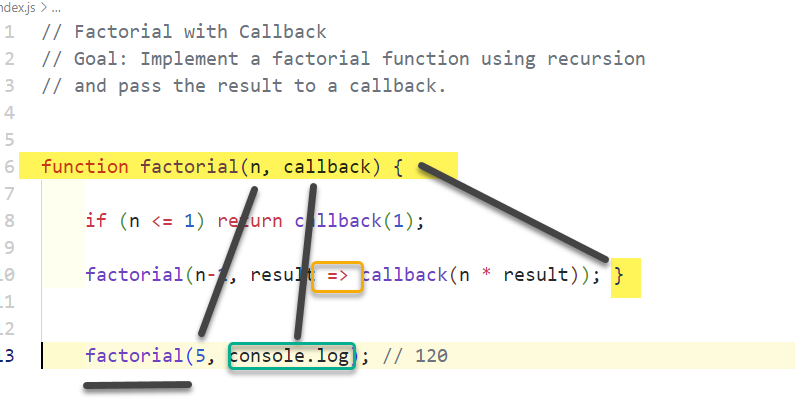Palindrome Checker with Callback
Goal: Create a function that checks if a given word is a palindrome.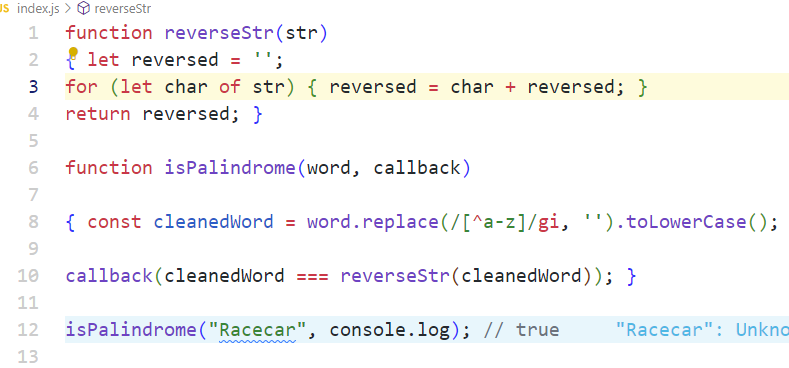Fibonacci Sequence with Promise
Goal: Generate the Fibonacci sequence up to the n-th term using recursion.
function fibonacci(n) { return new Promise((resolve, reject) => { if (n < 0) reject("Invalid input"); if (n <= 1) return resolve(n);
Promise.all([fibonacci(n-1), fibonacci(n-2)]) .then(([a, b]) => resolve(a + b)) .catch(reject); }); }
fibonacci(5).then(console.log); // 5
When n is less than or equal to 1, we can instantly resolve the promise with n.
For values of n greater than 1, we use Promise.all to wait for both recursive promises (fibonacci(n-1) and fibonacci(n-2)) to resolve.
Once both promises have resolved, we sum their results and then resolve the current promise with that sum.
This will give the correct result for fibonacci(5). However, note that this approach, while showcasing the combination of promises and recursion, is inefficient for larger values of n due to the exponential growth in the number of recursive calls. A more efficient solution would be to use an iterative approach or memoization with the recursive approach.
Let's break down the code line by line, focusing particularly on the operation of resolve and reject.
`javascriptCopy code``function fibonacci(n) {`
Here, we declare a function named fibonacci that takes a single argument, n, which represents the term number for which we want to find the Fibonacci value.
`javascriptCopy code`` return new Promise((resolve, reject) => {`
We're returning a new Promise object. A promise in JavaScript represents a value which might be available now, or in the future, or never. The promise constructor takes one argument: a function (often called the "executor function") with two parameters, resolve and reject, which are both functions.
The resolve function is used to signal the successful completion of the promise. When you call resolve, the promise's status changes from "pending" to "fulfilled", and any .then chained to the promise will execute its callback.
The reject function is used to signal that the promise has failed for some reason. When you call reject, the promise's status changes from "pending" to "rejected", and any .catch chained to the promise will execute its callback.
`javascriptCopy code`` if (n < 0) reject("Invalid input");`
This line checks if n is negative. If it is, the promise is immediately rejected with the reason "Invalid input". This means that the promise has failed and won't provide a valid Fibonacci number.
`javascriptCopy code`` if (n <= 1) return resolve(n);`
This is a base case for our recursive function. If n is 0 or 1, the Fibonacci value is equal to n. So, we immediately resolve the promise with n and return. This stops further execution within the executor function.
`javascriptCopy code`` Promise.all([fibonacci(n-1), fibonacci(n-2)])`
For values of n greater than 1, we're calculating the Fibonacci sequence using recursion. Here, we're making two recursive calls to fibonacci(n-1) and fibonacci(n-2), each of which returns a promise. Promise.all is a method that takes an array of promises and returns a new promise that only resolves when all of the input promises have resolved. If any of the input promises reject, the output promise is immediately rejected.
`javascriptCopy code`` .then(([a, b]) => resolve(a + b))`
If both promises (from the recursive calls) successfully resolve, their results are available as an array in the .then method. We destructure the results into a and b and then resolve our main promise with their sum, which is the Fibonacci value for term n.
`javascriptCopy code`` .catch(reject);`
If either of the recursive calls results in a rejected promise (which, in this specific scenario, shouldn't happen unless n is negative), we directly reject our main promise with the reason provided by the failed promise.
`javascriptCopy code`` });``}`
This closes our executor function and the fibonacci function definition.
`javascriptCopy code``fibonacci(5).then(console.log); // 5`
Lastly, we're calling the fibonacci function with an argument of 5 and attaching a .then method to log the result. If the promise resolves successfully, we'll log the resolved value (in this case, the Fibonacci value for term 5).

Flatten Array
Goal: Write a function that takes in a multi-dimensional array and returns a flattened version of it.
javascriptCopy code
function flatten(arr) { return arr.reduce((flat, item) => { return flat.concat(Array.isArray(item) ? flatten(item) : item); }, []); }
Debounce with Callback
Goal: Implement a debounce function.
javascriptCopy code
function debounce(fn, delay) { let timer; return function(...args) { clearTimeout(timer); timer = setTimeout(() => fn.apply(this, args), delay); } } const debouncedLog = debounce((text) => console.log(text), 1000); debouncedLog("Test");
Promise Chaining
Goal: Write a function that squares a number after a delay, using promises.
javascriptCopy code
function delayedSquare(n) { return new Promise((resolve) => { setTimeout(() => { resolve(n * n); }, 1000); }); } delayedSquare(5).then(console.log); // 25
Error Handling with Promises
Goal: Create a function that returns a promise which rejects after a delay.
javascriptCopy code
function failAfterDelay(delay) { return new Promise((resolve, reject) => { setTimeout(() => reject("Failed!"), delay); }); } failAfterDelay(1000).catch(console.error); // "Failed!"
Fetching Data using Promises
Goal: Fetch data from an API using promises.
`javascriptCopy code``function fetchData(url) {`` return fetch(url).then(response => {`` if (!response.ok) throw new Error("Network error");`` return response.json();`` });``}``fetchData("https://api.example.com/data").then(console.log).catch(console.error);`
I hope these drills help you practice JavaScript, callbacks, and promises effectively!